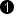## Operator ShiftLeft

The operator performs an arithmetic shift of the input data to the left. The number of bits to be shifted is defined using parameter Shift. Each bit at the input is left shifted by the parameterized number of bits. The newly inserted least significant bits will have value zero.

Bit shifting is usually used to scale a value by a power of two value. For example a left shift by one bit will double the value. A left shift by 2 bits will quadruple the input value.

Each color component is shifted separately.

### I/O Properties

Property Value
Operator Type O

Bit Width [1, 64] unsigned, [2, 64] signed autoArithmetic {unsigned, signed} as I
Parallelism any as I
Kernel Columns any as I
Kernel Rows any as I
Img Protocol {VALT_IMAGE2D, VALT_LINE1D, VALT_PIXEL0D} as I
Color Format any as I
Color Flavor any as I
Max. Img Width any as I
Max. Img Height any as I
 The bit width at the output is the input bit width plus the value of parameter Shift.

### Parameters

Shift
Type static parameter
Default 0
Range [0, 64 - Input BitWidth]

This parameter defines the number of bits by which the input is left shifted.

### Examples of Use

The use of operator ShiftLeft is shown in the following examples: